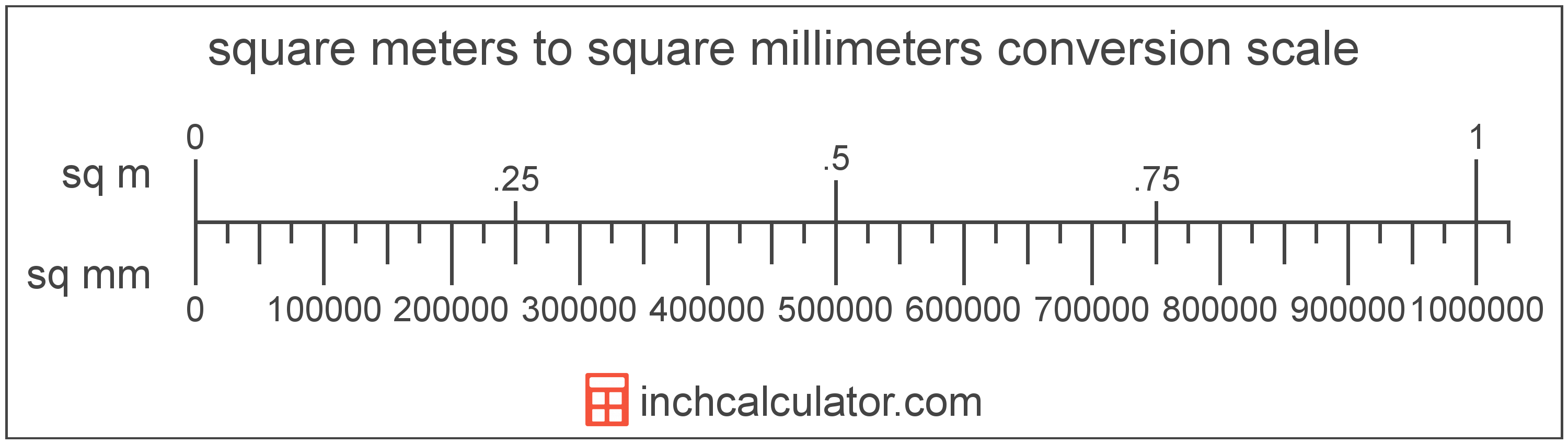# Square Millimeters to Square Meters Converter

Enter the area in square millimeters below to get the value converted to square meters.

Results in Square Meters:1 sq mm = 1.0E-6 sq m
Hint: use a scientific notation calculator to convert E notation to decimal

Do you want to convert square meters to square millimeters?

## How to Convert Square Millimeters to Square Meters

To convert a measurement in square millimeters to a measurement in square meters, divide the area by the following conversion ratio: 1,000,000 square millimeters/square meter.

Since one square meter is equal to 1,000,000 square millimeters, you can use this simple formula to convert:

square meters = square millimeters ÷ 1,000,000

The area in square meters is equal to the area in square millimeters divided by 1,000,000.

For example, here's how to convert 5,000 square millimeters to square meters using the formula above.
square meters = (5,000 sq mm ÷ 1,000,000) = 0.005 sq m## What is a Square Millimeter?

One square millimeter is equivalent to the area of a square with edges that are each 1 millimeter in length. One square millimeter is equal to 0.00155 square inches or 1/100 of a square centimeter.

The square millimeter, or square millimetre, is a multiple of the square meter, which is the SI derived unit for area. In the metric system, "milli" is the prefix for thousandths, or 10-3. A square millimeter is sometimes also referred to as a square mm. Square millimeters can be abbreviated as sq mm, and are also sometimes abbreviated as mm². For example, 1 square millimeter can be written as 1 sq mm or 1 mm².

## What is a Square Meter?

One square meter is equivalent to the area of a square with sides that are each 1 meter in length. One square meter is equal to 10.76391 square feet or 10,000 square centimeters.

The square meter, or square metre, is the SI derived unit for area in the metric system. A square meter is sometimes also referred to as a square m. Square meters can be abbreviated as sq m, and are also sometimes abbreviated as . For example, 1 square meter can be written as 1 sq m or 1 m².

You can use a square meters calculator to calculate the area of a space if you know its dimensions.

## Square Millimeter to Square Meter Conversion Table

Table showing various square millimeter measurements converted to square meters.
Square Millimeters Square Meters
1 sq mm 0.000001 sq m
2 sq mm 0.000002 sq m
3 sq mm 0.000003 sq m
4 sq mm 0.000004 sq m
5 sq mm 0.000005 sq m
6 sq mm 0.000006 sq m
7 sq mm 0.000007 sq m
8 sq mm 0.000008 sq m
9 sq mm 0.000009 sq m
10 sq mm 0.00001 sq m
100 sq mm 0.0001 sq m
1,000 sq mm 0.001 sq m
10,000 sq mm 0.01 sq m
100,000 sq mm 0.1 sq m
1,000,000 sq mm 1 sq m

## References

1. Collins Dictionary, Definition of 'square meter', https://www.collinsdictionary.com/us/dictionary/english/square-meter